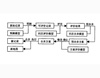• 详细介绍了模糊综合评价法的案例与运用，特别是旅游专业的学生会比较适用
• 模糊综合评价法原理及案例分析，模糊综合评价法原理及案例分析课件，模糊综合评价法原理及案例分析PPT
• 主要研究了采用模糊综合评价法对煤矿机械制造工艺方案进行选择。首先介绍了模糊综合评价法,然后结合例子介绍了煤矿机械制造工艺方案的确定,最后阐述了以模糊综合评价法为原则的煤矿机械制造工艺方案评价体系的建立和...
• 文章首先分析了煤炭开采对各环境要素产生的影响,根据煤矿在开采过程中对水、大气、土地等环境要素的影响选取了...最后,基于模糊综合评价法,结合具体的案例,通过计算指标权重矩阵和模糊评价矩阵,得出煤矿环境综合评分。
• 利用二级模糊综合评价法构建企业管理能力的综合评价模型，游雪，陈欢，本文通过二级模糊综合评价法，给出构建企业管理能力评价的模糊综合评价模型的步骤，并通过实例进行说明，实现了对企业管理能力的
• 教师质量评价，模糊综合评价法，综合评价分析跟研究
• 利用群决策层次分析法和因子分析法分别确定主客观权重,再将两者集结的综合权重作为模型各层因素的权重,最后利用改进的模糊综合评价法完成模型评价工作。选取郑州某煤矿为实证研究对象,对其进行实地调研、问卷调查和...
• 基于模糊综合评价法的旅游资源评价研究，冯霞，，模糊综合评判法是对难以定量研究的事物进行量化研究的有益尝试，取得的结果较有说服力。本文在理解模糊综合评判法的内涵的基础上
• 模糊综合评价法在空气质量评价中的应用，尹玲，赵婷婷，传统的评价方法不能处理带有模糊信息的空气质量评价问题，空气的污染程度具有模糊概念，需要用模糊理论进行研究。模糊综合评价法
• 在建立煤矿安全文化评价模型的基础上,运用AHP法确定各个评价指标的权重,最后将模糊综合评价法应用到煤矿安全文化评价中。根据评价结果,山西某煤矿企业安全文化所属等级为团队互助阶段。实践证明,该方法可操作性强,...
• 分析了煤矿职业危害的三类危险源,构建了基于层次分析法的煤矿职业危害危险源风险评价模型,运用模糊综合评价法对3个煤矿的职业危害危险源进行了风险评价,评价结果合理可靠。煤矿职业危害风险评价研究对于丰富职业危害...
• 从煤矿"六大系统"验收标准的角度出发,运用模糊综合评价法构建了煤矿"六大系统"评价指标体系,并将该评价指标体系分成若干个一级指标、二级指标以及否决项,利用层次分析法确定评价因素的权重,实现了煤矿"六大系统"质量...
• 文章结合煤矿安全生产的实际情况,分析了影响煤矿安全生产的各个因素集,利用层次分析法和模糊综合评价法建立了煤矿生产安全评价体系的数学模型。将该数学模型应用在煤矿企业安全生产评价及安全资金投入分配工作中,...
• 模糊综合评价法能反映水质评价中的模糊概念，是客观评价目标水质的一种常用水质评价方法。但在传统的模糊综合评价法中，多采用“超标法”计算权重，为了避免超标法计算权重的不合理之处，笔者分别用“改进超标法”和...
• 基于模糊综合评价法的纯净水安全风险评价，盛蒙蒙，揭宗昌，本文针对纯净水安全问题，建立了纯净水安全风险评价模型，运用模糊综合评价的方法对纯净水的各个风险因素进行分析，通过风险度定
• 尽管我国煤矿安全管理水平在不断提高,但是我国煤矿的安全形势依然非常严峻,因此需要...然后,结合具体的案例,基于专家打分法和模糊综合评价法,计算得出模糊评价矩阵,产生煤矿安全风险综合评分,最终界定煤矿的安全等级。
• 模糊综合评价法的特点在于，评价对象逐个进行，对评价对象有唯一的评价值，不受评价对象所处对象集合的影响。 模糊综合评价的数学模型分为一级模型和多级模型，一级模型也称为单层次评价模型。首先对影响总目标的多...
一、简介
模糊综合评价是以模糊数学为基础，应用模糊关系合成的原理，将一些边界不清，不易定量的因素定量化，进行综合评价的一种方法
例如“年轻”与“年老”、“高”与“矮”、“欢迎”与“不欢迎”等等。凡是涉及到模糊概念的现象，即称之为模糊现象。
模糊综合评价法的特点在于，评价对象逐个进行，对评价对象有唯一的评价值，不受评价对象所处对象集合的影响。
模糊综合评价的数学模型分为一级模型和多级模型，一级模型也称为单层次评价模型。首先对影响总目标的多个因素列出构成一个因素集(评价指标)，同时建立评价集(评价等级)。然后进行单因素评判，根据评价集对因素集中每一个因素进行模糊判断，确定每个因素在评价集中对各个评价等级的隶属度的大小，构成模糊矩阵。对因素集中每个因素赋予不同的权重，构成权重向量，从而得出最后的评判结果。
二、模糊综合评价法优缺点
（1）优点

模糊综合评价法通过精确的数字手段处理模糊的评价对象，数学模型简单，容易掌握；
可以将不完全信息、不确定信息转化为模糊概念，使定性问题定量化，提高评估的准确性；
评价结果可以是一组向量，也可以是一个点值，包含的信息比较丰富。

（2）缺点

模糊关系矩阵、权重、算子的选择都有一定的主观性；
隶属函数的确定还没有明确的且系统的方法；
当同一指标层级的指标个数较多时，会导致权系数较小，出现超模糊现象，分辨率较差，造成评估失败。

三、模糊综合评价方法和步骤
模糊综合评价是通过构造等级模糊子集把反映被评事物的模糊指标进行量化(即确定隶属度)，然后利用模糊变换原理对各指标综合
（1）确定评价对象的因素域值，即建立指标集
基于评价指标体系
例如

X
一级指标
二级指标

X1
X11

X12

X13

X2
X21

X22

X23

X3
X31

X32

设$X=\{X_1,X_2,\cdots ,X_m\}$为待评价对象的 m 种指标，称为第一级评价指标集。
依据第一级评价指标集的具体情况，设定下属的第二级评价指标集
$X_i=\{X_{i1},X_{i2},\cdots ,X_{it}\}$，其中i=1,2,…,m。
依次类推，如需要，还可设定下属的第三级、第四级评价指标集，在此只研究到第二级的评价指标集。
（2）确定评语集
设$Y=\{Y_1,Y_2,\cdots ,Y_n\}$为 n 种评语或等级
评语等级是模糊综合评价所必备的要素，必须事先进行确定。评语等级是用来对各个因素进行评价的模糊概念，评语集合是对评价对象作出各种不同评价的一个集合，集合的确定能够使得模糊综合评价得到一个模糊评价向量，通过评语的等级隶属度显示出来，
例如
选择评价等级为5，即设$Y=\{Y_1,Y_2,\cdots ,Y_5\}$ ，其中Y表示评语集，$Y_1$表示很满意，$Y_2$表示满意， $Y_3$表示一般， $Y_4$表示不太满意， $Y_5$表示很不满意。不同的满意评价代表不同的满意状况
（3）建立模糊关系矩阵R
评价等级设置好之后，接下来就要判断X的每个因素相对于评价等级的隶属程度，隶属程度指的是某一项指标对于某一评价等级的属于程度。进而得到模糊关系矩阵
$R=\left( \begin{array}{c} \begin{matrix} R|& \text{x}_1\\ R|& x_2\\ \end{matrix}\\ \begin{array}{c} \cdots\\ \begin{matrix} R|& x_m\\ \end{matrix}\\ \end{array}\\ \end{array} \right) =\left( \begin{array}{c} r_{11}\\ r_{12}\\ \vdots\\ r_{m1}\\ \end{array}\begin{array}{c} \\ \\ \\ \end{array}\begin{array}{c} r_{12}\\ r_{22}\\ \vdots\\ r_{m2}\\ \end{array}\begin{array}{c} \\ \\ \\ \end{array}\begin{array}{c} \cdots\\ \cdots\\ \vdots\\ \cdots\\ \end{array}\begin{array}{c} \\ \\ \\ \end{array}\begin{array}{c} r_{1n}\\ r_{2n}\\ \vdots\\ r_{mn}\\ \end{array} \right) _{mn}$
其中m表示指标数，n表示评价等级，$r_{ij}$表示因素$X_i$对模糊子集（评语）$Y_j$的隶属度。一般将其归一化处理，使得矩阵行和等于1，即
$\sum_j^n{r_{ij}}=1$
一个被评事物在某个因素$X_i$方面的表现，是通过模糊向量
$\left( R|x_i \right) =\left( r_{i1},r_{i2},\cdots \cdots ,r_{in} \right)$
来刻画的，而在其他评价方法中多是由一个指标实际值来刻画的，因此，从这个角度讲模糊综合评价要求更多的信息
也就是说
在指标集（因素集）中存在某个因素$X_i$，i=1,2,…,m，该因素对评语集Y的隶属向量度为$r_i=\left( r_{i1},r_{i2},\cdots ,r_{in} \right)$，其中 n 表示 n 种评语或等级，比如上面提到的$Y=\{Y_1,Y_2,\cdots ,Y_5\}$ ，此时 n=5。
同理，对于全部因素，都可以构造出与因素对应的隶属度向量，将其组成隶属向量矩阵R
对于模糊评判矩阵的构造，一般有两种情况：第一种是对于主观或者定性指标的模糊评判矩阵构造，可以使用等级比重法；第二种是对于客观或定量指标的模糊评判矩阵的构造，可以使用频率法。
（4）确定指标权重
在模糊综合评价中，确定评价因素的权向量$W=\left( w_1,w_2,\cdots ,w_m \right)$
权向量W中的元素$w_i$本质上是因素$X_i$对模糊子(对被评事物重要的因素)的隶属度。
在模糊综合评价法中，权重的确定一般需要专家的知识和经验，具有一定的缺陷。通常采用层次分析法来确定各指标的权系数，使其更有合理性，更符合客观实际并易于定量表示，从而提高模糊综合评判结果的准确性
（5）建立一级指标模糊评价
一级模糊评价的建立需要利用模糊合成算子来进行，合成过程如下：
$W\circ R=\left( w_1,w_2,...w_m \right) \circ \left[ \begin{array}{c} r_{11}\\ r_{12}\\ \vdots\\ r_{m1}\\ \end{array}\begin{array}{c} \\ \\ \\ \end{array}\begin{array}{c} r_{12}\\ r_{22}\\ \vdots\\ r_{m2}\\ \end{array}\begin{array}{c} \\ \\ \\ \end{array}\begin{array}{c} \cdots\\ \cdots\\ \vdots\\ \cdots\\ \end{array}\begin{array}{c} \\ \\ \\ \end{array}\begin{array}{c} r_{1n}\\ r_{2n}\\ \vdots\\ r_{mn}\\ \end{array} \right] =\left( \text{b}_1,b_2,\cdots ,\text{b}_n \right) \triangleq B$
其中“$\circ$” 为模糊合成算子，$B=\left( b_1,b_2,\cdots ,b_n \right)$为模糊评价结果向量，一般需要归一化处理，满足
$\sum_j^{}{b_j}=1$
一般模糊合成算子有四种：
$M\left( \land ,\lor \right) \text{，}M\left( \bullet ,\lor \right) \text{，}M\left( \bullet ,\oplus \right) \text{，}M\left( \land ,\oplus \right)$
举个简单例子
$B\ =\ W\circ R=\left( 2,0,1 \right) \left( \begin{matrix} 1& 2& 0\\ 3& 1& 1\\ 0& 4& 2\\ \end{matrix} \right)$

$M\left( \land ,\lor \right)$ 表示先取小，再取大。
先取小：
左边第一个与右边第一列的第一个（2和1—取1）
左边第二个与右边第一列的第二个（0和3—取0）
左边第三个与右边第一列的第三个（1和0—取0）
再取大：
（1,0,0）-----取1
同理
（2,0,1）-----取2
（0,0,1）-----取1
最终：

$B\ =\ W\circ R=\left( 2,0,1 \right) \left( \begin{matrix} 1& 2& 0\\ 3& 1& 1\\ 0& 4& 2\\ \end{matrix} \right) =\left( 1,2,1 \right)$

$M\left( \bullet ,\lor \right)$ 表示先乘，再取大
同上边的计算方法一样，不同的只在于上边是先取小，这里是先相乘
结果为：

$B\,\,=\,\,W\circ R=\left( 2,0,1 \right) \left( \begin{matrix} 1& 2& 0\\ 3& 1& 1\\ 0& 4& 2\\ \end{matrix} \right) =\left( 2,4,2 \right)$

$M\left( \bullet ,\oplus \right)$ 表示先相乘，再求和
结果为：
$B\,\,=\,\,W\circ R=\left( 2,0,1 \right) \left( \begin{matrix} 1& 2& 0\\ 3& 1& 1\\ 0& 4& 2\\ \end{matrix} \right) =\left( 2,8,2 \right)$

$M\left( \land ,\oplus \right)$表示先取小，再求和
结果为：

$B\,\,=\,\,W\circ R=\left( 2,0,1 \right) \left( \begin{matrix} 1& 2& 0\\ 3& 1& 1\\ 0& 4& 2\\ \end{matrix} \right) =\left( 1,3,1 \right)$
最后，在实际应用中  $\left( \land ,\lor \right) \text{，}\left( \bullet ,\oplus \right)$算子最为常用。
四种模糊合成算子的特点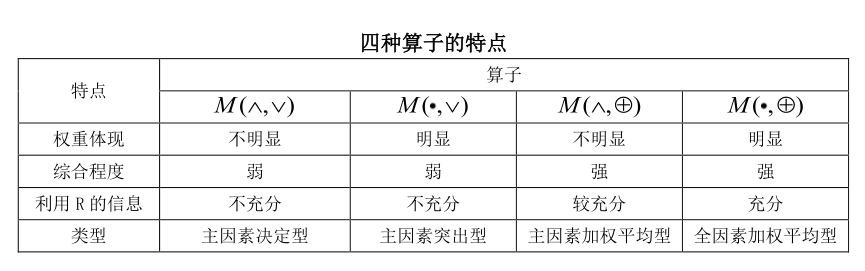（6）建立二级模糊评价
根据以上一级模糊综合评价的结果，作为二级模糊综合评价的关系矩阵，利用前述方法，进一步得到二级模糊综合评价结果。
（7） 模糊评价结果处理
模糊综合评价法中提供了两种可供选择的结果处理方法，这里采用参数加权平均法进行结果的处理计算，具体计算过程如下：
在评判向量$B\left( b_1,b_2,\cdots ,b_j,\cdots ,b_n \right)$中给各评定等级以参数值 $c_1,c_2...,c_k$，等级由高到低，且间距相等，为了突出优势等级的作用，可用各隶属度的幂加权平均法得到参数加权平均的公式：
$C=\frac{\sum\limits_{j=1}^n{b_{j}^{t}c_k}}{\sum\limits_{j=1}^n{b_{j}^{t}}}$
其中，t为待定系数，根据具体情况而定，一般可取 t=1,2
最后根据C的取值，根据评价定量分级标准对用户的满意度进行评级，对照表如下：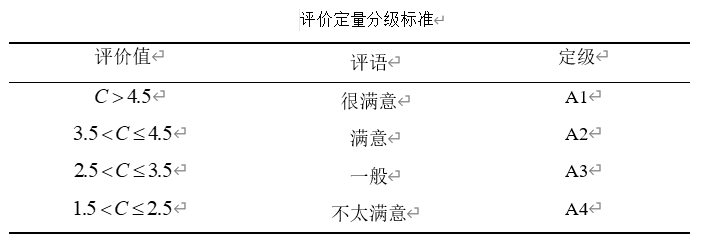补充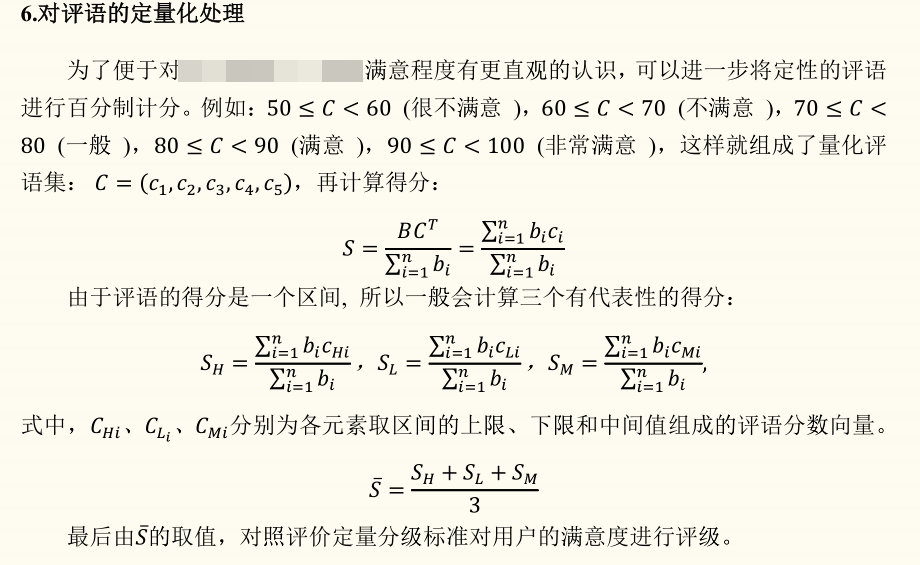两种定量化处理都可以
例子1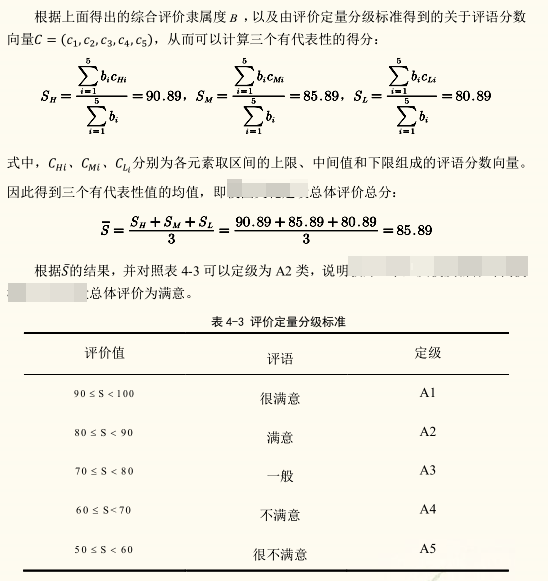例子2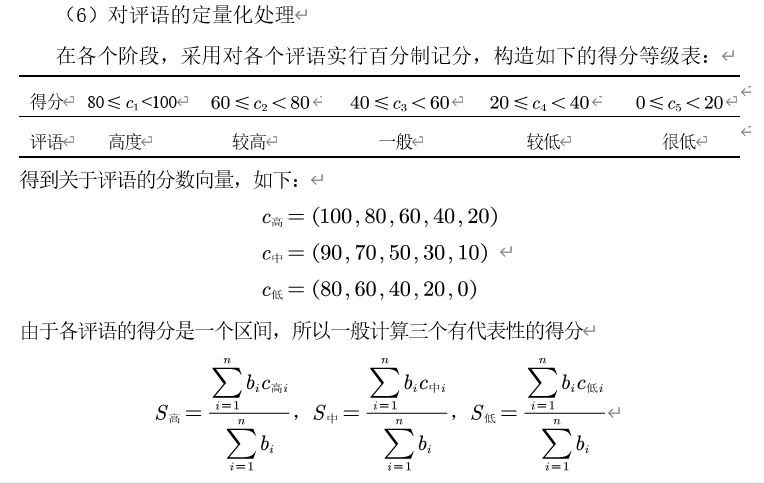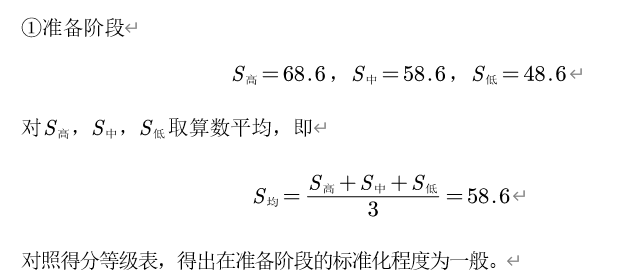最后
模糊综合评价也可以使用软件完成
http://note.youdao.com/noteshare?id=2d89910d18eeb75d77d99737b0eb7d3d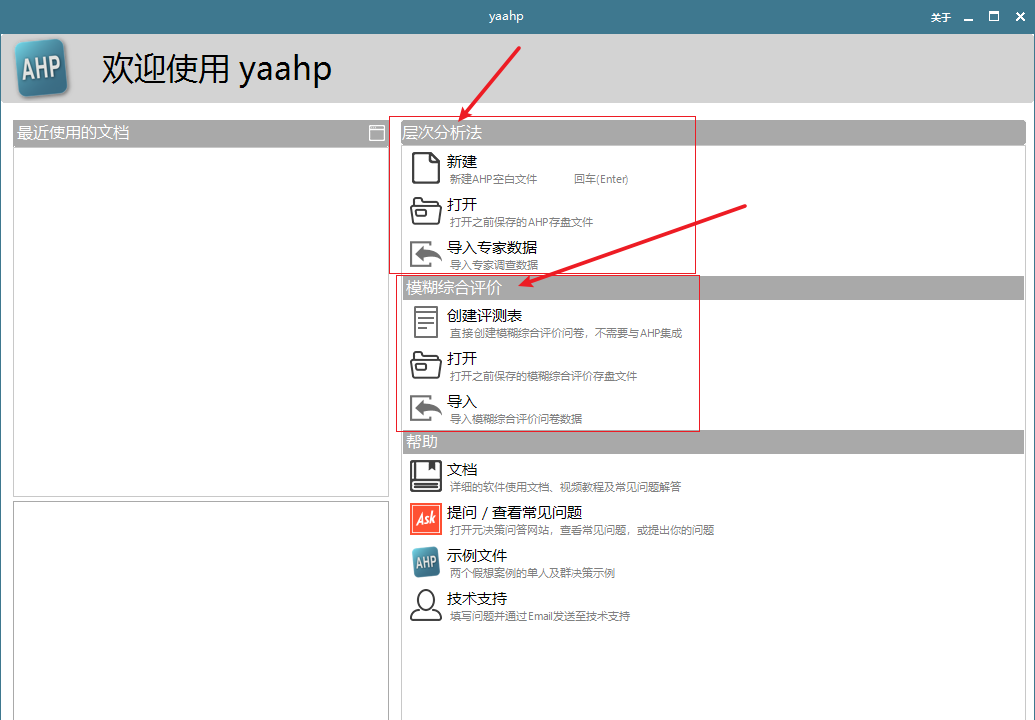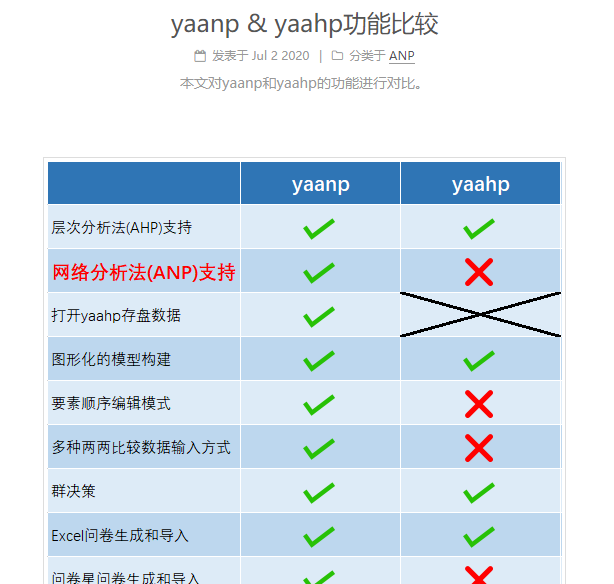python实现
请参见博文：
模糊层次综合分析法Python实践及相关优缺点分析


展开全文• 本文采用模糊综合评价法对杨庄煤矿地质构造因素进行定量评价,通过构建各地质构造因素的隶属函数,结合各因素权重计算,按照最大隶属度原则得出结论,认为该矿地质构造类型为复杂。
• 常见的模糊综合评价法.ppt-原创力文档 (book118.com)
常见的模糊综合评价法.ppt-原创力文档 (book118.com)

模糊综合评价法原理及案例分析 - 豆丁网 (docin.com)

基于层次分析法的模糊综合评价 - 豆丁网 (docin.com)

SPSS在线_SPSSAU_SPSS_模糊综合评价


展开全文• 模糊综合评价法在研究生教育评价中的应用，赵英男，，研究生教育问题现今已成为社会各界人士关注的焦点。文章首先建立了研究生教育评价指标体系，并在此基础上采用了模糊综合评价方法
• 为了确定矸石山绿化方向,利用模糊综合评价法对其适宜性进行评价。根据模糊综合评价原理,建立矸石山绿化评价模型。选取影响评判矸石山绿化的因素(坡度、地表组成物质、覆土厚度、灌溉条件、排水条件、土壤肥力)为评价...
• 模糊综合评价法在烟气脱硫技术选择中的应用，周小力，唐佳明，针对烟气脱硫技术种类多，技术、经济等指标繁杂，用户不容易选取的特点，引入模糊综合评价法。对几种有代表性的烟气脱硫技术进行
• 基于模糊综合评价法的LIMS界面设计，郑斐，沙菁㛃，实验室信息管理系统（LIMS）发展愈加迅速，但针对系统界面的设计研究仍然缺乏。本文以某省重点实验室为例，给出了LIMS界面设计的方�
• 随着工业社会向信息社会转变，企业经营管理的内容和重点发生了很大的变化，传统的以财务绩效评价为主要...通过杜邦财务分析体系、沃尔评分法分析了企业财务综合状况，再运用模糊综合评价法对企业绩效进行了综合评价。
• 运用模糊综合评价法研究城市火灾风险的评价问题，提出运用模糊综合评判法研究城市火灾风险的方法和步骤，并应用于实践。
• 精品文档可编辑 值得下载 基于模糊综合评价法的网络组织竞争力分析 摘要本文通过对国内外文献的研究将影响网络组织竞争力的内外部因素作为评价指标在此基础上通过收集相关数据运用层次分析法来确定各项指标的权重......